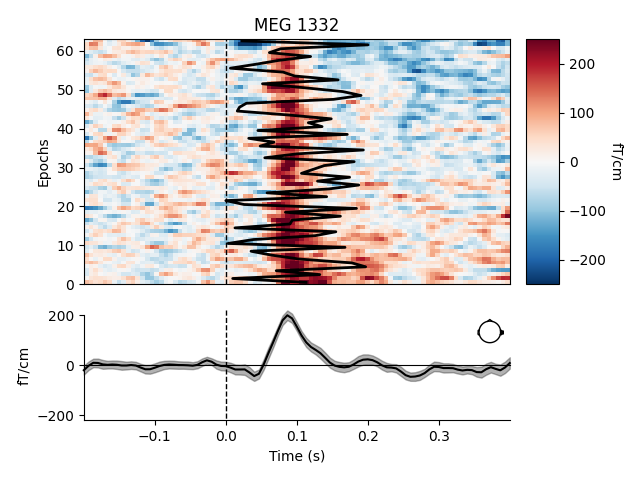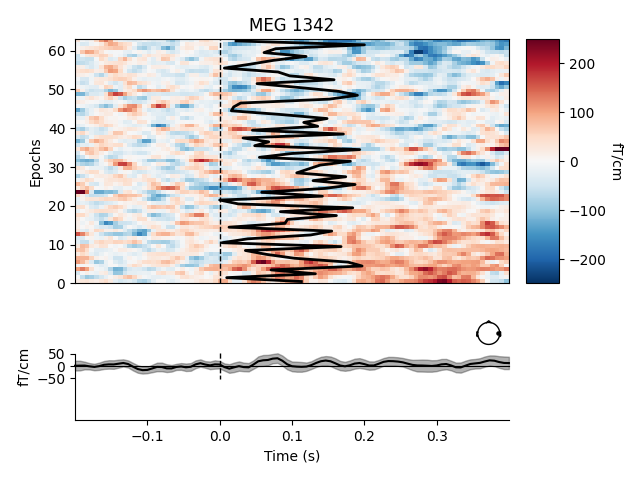# Visualize channel over epochs as an image¶

This will produce what is sometimes called an event related potential / field (ERP/ERF) image.

Two images are produced, one with a good channel and one with a channel that does not show any evoked field.

It is also demonstrated how to reorder the epochs using a 1D spectral embedding as described in 1.

```# Authors: Alexandre Gramfort <alexandre.gramfort@inria.fr>
#

import numpy as np
import matplotlib.pyplot as plt

import mne
from mne import io
from mne.datasets import sample

print(__doc__)

data_path = sample.data_path()
```

Set parameters

```raw_fname = data_path + '/MEG/sample/sample_audvis_filt-0-40_raw.fif'
event_fname = data_path + '/MEG/sample/sample_audvis_filt-0-40_raw-eve.fif'
event_id, tmin, tmax = 1, -0.2, 0.4

# Setup for reading the raw data

# Set up pick list: EEG + MEG - bad channels (modify to your needs)
raw.info['bads'] = ['MEG 2443', 'EEG 053']

# Create epochs, here for gradiometers + EOG only for simplicity
epochs = mne.Epochs(raw, events, event_id, tmin, tmax, proj=True,
```

Out:

```Opening raw data file /home/circleci/mne_data/MNE-sample-data/MEG/sample/sample_audvis_filt-0-40_raw.fif...
Read a total of 4 projection items:
PCA-v1 (1 x 102)  idle
PCA-v2 (1 x 102)  idle
PCA-v3 (1 x 102)  idle
Average EEG reference (1 x 60)  idle
Range : 6450 ... 48149 =     42.956 ...   320.665 secs
72 matching events found
Setting baseline interval to [-0.19979521315838786, 0.0] sec
Applying baseline correction (mode: mean)
4 projection items activated
Rejecting  epoch based on EOG : ['EOG 061']
Rejecting  epoch based on EOG : ['EOG 061']
Rejecting  epoch based on EOG : ['EOG 061']
Rejecting  epoch based on EOG : ['EOG 061']
Rejecting  epoch based on EOG : ['EOG 061']
Rejecting  epoch based on EOG : ['EOG 061']
Rejecting  epoch based on EOG : ['EOG 061']
Rejecting  epoch based on EOG : ['EOG 061']
Rejecting  epoch based on EOG : ['EOG 061']
```

Show event-related fields images

```# and order with spectral reordering
# If you don't have scikit-learn installed set order_func to None
from sklearn.manifold import spectral_embedding  # noqa
from sklearn.metrics.pairwise import rbf_kernel   # noqa

def order_func(times, data):
this_data = data[:, (times > 0.0) & (times < 0.350)]
this_data /= np.sqrt(np.sum(this_data ** 2, axis=1))[:, np.newaxis]
return np.argsort(spectral_embedding(rbf_kernel(this_data, gamma=1.),
n_components=1, random_state=0).ravel())

good_pick = 97  # channel with a clear evoked response
bad_pick = 98  # channel with no evoked response

# We'll also plot a sample time onset for each trial
plt_times = np.linspace(0, .2, len(epochs))

plt.close('all')
order=order_func, vmin=-250, vmax=250,
overlay_times=plt_times, show=True)
```
••Out:

```Not setting metadata